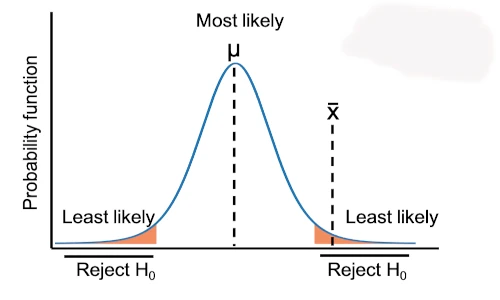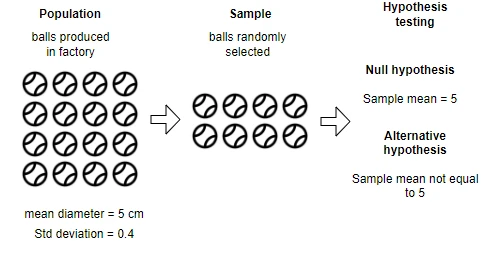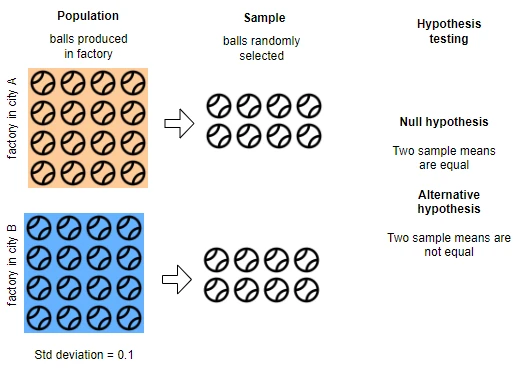# Analyze one and two-sample Z-test in RIn this article, you will learn how to perform three types of Z tests (one sample, two samples, and paired Z test) in R. If you would like to learn more about Z test background, hypotheses, formula, and assumptions, you can refer to this detailed article on how to perform Z-test in Python.

## 1. One Sample Z-test

One sample Z-test compares the sample mean with a specific hypothesized mean of the population. Unlike t-test, the mean and standard deviation of the population should be known in the Z-test.

Example: A factory produces balls of a diameter of 5 cm. But due to manufacturing conditions, every ball may not have the exactly same diameter. The standard deviation of the diameters of balls is 0.4. Now, the quality officer would like to test whether the ball diameter is significantly different from 5 cm in a sample of 50 balls randomly taken from the manufacturing line.Import dataset for testing ball diameter using Z-test,

Read more here how to import CSV dataset in R

df = read.csv('https://reneshbedre.github.io/assets/posts/ztest/z_one_samp.csv')
# output
sizes
1 4.819289
2 3.569358

# determine the size of data frame
dim(df)
# output
 50  1


Now, perform one sample Z-test using the z.test() function available in the BSDA package. z.test() function takes the following required arguments,

x: Numeric vector for one sample data (use this in case of one sample Z-test)
mu: hypothesized or known population mean (specified in null hypothesis)
alternative: Type of test to calculate p value (two-sided, greater, or less). By default, it performs two-sided test.
sigma.x: population standard deviation for x

library(BSDA)

# perform two-sided test
z.test(x = df, mu = 5, sigma.x = 0.4)
# output
One-sample z-Test

data:  df
z = 0.31746, p-value = 0.7509
alternative hypothesis: true mean is not equal to 5
95 percent confidence interval:
4.907086 5.128831
sample estimates:
mean of x
5.017959


If you want to perform one-sided test, then add an option alternative: 'greater' or alternative: 'less' based on the hypothesis.

#### Interpretation

As the p value of the one-sample Z-test is not significant [Z value = 0.3174, p = 0.7509], we conclude that the mean diameter of balls in a random sample is equal to the population mean of 5 cm. It is, therefore, a sample drawn from an identical population.

### 2.2 Two sample Z-test (unpaired or independent Z-test)

The two-sample (unpaired or independent) Z-test calculates if the means of two independent groups are equal or significantly different from each other. Unlike the t-test, Z-test is performed when the population means and standard deviation are known.

#### Calculate Two sample Z-test in R

Example: a factory in A and B cities produces balls of a diameter of 5 cm. The defect is reported in the B factory which is known to cause a change in ball size. The standard deviation of the diameters of the balls is 0.1. The factory quality officer randomly selects 50 balls from both factories to test whether the ball diameter is significantly different in factory B than that produced in factory A.Import dataset for testing ball diameter using two sample Z-test,

Read more here how to import CSV dataset in R

df = read.csv('https://reneshbedre.github.io/assets/posts/ztest/z_two_samp.csv')
# output
fact_A   fact_B
1 4.977904 5.887947
2 5.166254 5.990616

# determine the size of data frame
dim(df)
# output
 50  2


Now, perform one sample Z-test using the z.test() function available in the BSDA package. z.test() function takes the following required arguments,

x: Numeric vector for x group
y: Numeric vector for y group
sigma.x: population standard deviation for x group
sigma.y: population standard deviation for y group
alternative: Type of test to calculate p value (two-sided, greater, or less). By default, it performs two-sided test.

library(BSDA)

# perform two-sided test
z.test(x = df1$fact_A, y = df1$fact_B,  sigma.x = 0.1, sigma.y = 0.1)
# output
Two-sample z-Test

data:  df1$fact_A and df1$fact_B
z = -48.866, p-value < 2.2e-16
alternative hypothesis: true difference in means is not equal to 0
95 percent confidence interval:
-1.0165115 -0.9381129
sample estimates:
mean of x mean of y
5.012839  5.990151


#### Interpretation

Z-test results for the two samples show a highly significant p value [Z = -48.866, p < 0.001]. This indicates that the balls produced in factories A and B are significantly different in size. As a result, factory B’s ball production machine has a defect.

### 2.3 Paired Z-test (dependent Z-test)

• Paired Z-test is used for checking whether there is difference between the two paired samples or not. For example, we have plant variety A and would like to compare the yield of variety A before and after the application of fertilizer.

#### Calculate paired Z-test in R

Example: a researcher wants to test the effect of fertilizer on plant growth. The researcher measures the height of the plants before and after the application of fertilizer. The standard deviations for two plant height differences (before and after the application of fertilizer) is 1.2. A researcher wants to test if there is an increase in plant heights after the application of fertilizers.

Import dataset for paired Z-test,

Read more here how to import CSV dataset in R

df = read.csv('https://reneshbedre.github.io/assets/posts/ztest/paired_z.csv')
# output
before    after
1 75.73154 83.15463
2 75.27301 83.32258

dim(df)
# output
 60  2


If the assumed population difference is zero (as stated in the null hypothesis), the paired Z-test reduces to the one sample Z-test. Hence, we will perform one sample Z-test on paired differences.

library(BSDA)

# get difference
df$diff = df$after - df$before head(df, 2) # output before after diff 1 75.73154 83.15463 7.423096 2 75.27301 83.32258 8.049578 # perform one sample Z-test on differences (two-sided test) z.test(x = df$diff, mu = 0, sigma.x = 1.2)
# output
One-sample z-Test

data:  df\$diff
z = 67.278, p-value < 2.2e-16
alternative hypothesis: true mean is not equal to 0
95 percent confidence interval:
10.11903 10.72630
sample estimates:
mean of x
10.42267


#### Interpretation

Based on the paired Z-test, the p value is highly significant [Z = 67.278, p < 0.001]. The plant height increased significantly after fertilizer was applied. We conclude that the application of fertilizer significantly contributes to the height increase of plants.

## Enhance your skills with courses on Statistics and R

• Heumann C, Shalabh MS. Introduction to statistics and data analysis. Springer; 2016.

If you have any questions, comments or recommendations, please email me at reneshbe@gmail.com